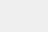# Python标准库time使用方式详解

小编写这篇文章的主要目的，是给大家进行一个解答，解答关于标准库times的使用方式一些，具体的操作，下面就给大家进行一个解答。

1、time库

时间戳(timestamp)的方式：通常来说，时间戳表示的是从1970年1月1日00:00:00开始按秒计算的偏移量

结构化时间(struct_time)方式：struct_time元组共有9个元素

格式化的时间字符串(format_string)，时间格式的字符串

1.1、获取格林威治西部的夏令时地区的偏移秒数

如果该地区在格林威治东部会返回负值（如西欧，包括英国）

对夏令时启用地区才能使用

```　#coding:utf-8
importtime
#获取格林威治西部的夏令时地区的偏移秒数。
print(time.altzone)```1.2、时间函数

时间戳

指格林威治时间1970年01月01日00时00分00秒(北京时间1970年01月01日08时00分00秒)起至现在的总秒数

时间元组

用一个元组装起来的9组数字```　#coding:utf-8
importtime
#返回当前时间的时间戳（1970纪元后经过的浮点秒数）
print(time.time())
#接收时间戳返回一个24个定长可读形式的字符串
print(time.ctime())#ThuJul720:17:142022
print(len(time.ctime()),type(time.ctime()))#24<class'str'>
#接收时间戳（1970纪元后经过的浮点秒数）并返回当地时间下的时间元组
print(time.localtime())
print(time.localtime(time.time()))
#接收时间戳（1970纪元后经过的浮点秒数）并返回格林威治天文时间下的时间元组
print(time.gmtime())
print(time.gmtime(time.time()))
#接收时间元组并返回一个可读的形式为"ThuJul720:11:042022"（2022年7月7日周四20时11分04秒）的字符串，长度固定为24字符
print(time.asctime())
print(time.asctime(time.gmtime()))
print(time.asctime(time.localtime()))
print(len(time.asctime()),type(time.asctime()))#24<class'str'>
#接受时间元组并返回时间戳（1970纪元后经过的浮点秒数）
#print(time.mktime())#参数不可为空TypeError:time.mktime()takesexactlyoneargument(0given)
print(time.mktime(time.gmtime()))
print(time.mktime(time.localtime()))
print(type(time.mktime(time.gmtime())))#浮点秒数<class'float'>
#返回以秒为单位的时间浮点值
print(time.perf_counter())
print(type(time.perf_counter()))
#返回以纳秒为单位的时间整数值
print(time.perf_counter_ns())#<class'float'>
print(type(time.perf_counter_ns()))#<class'int'>
1.3、格式化时间、日期
时间格式，格式化日期和时间时使用
#coding:utf-8
importtime
importdatetime
#格式化日期
print(time.strftime('%Y-%m-%d%H:%M:%S'))
print(time.strftime('%Y-%m-%d%I:%M:%S'))
print(time.strftime('%X'))
print(time.strftime('%Z'))
#将字符串转时间元组
#'%a%b%d%H:%M:%S%Y'
print(time.strptime('2022-07-0709:58:24','%Y-%m-%d%H:%M:%S'))
print(time.strptime('2022-07-0709:58:24','%Y-%m-%d%I:%M:%S'))```1.4、单调时钟

单调时钟是不能向后移动的时钟

常用来计算程序运行处理时长

```　　#coding:utf-8
importtime
#获取单调时钟的值,单调时钟是不能向后移动的时钟
#常用来计算程序运行处理时长
start_time=time.monotonic()
i=0
print(f"循环开始时钟值：{start_time}",type(start_time))#循环开始时钟值：6718828000000<class'int'>
whilei<=10:
time.sleep(0.1)
i+=1
end_time=time.monotonic()
print(f"循环结束时钟值：{end_time}")
print(f"循环运行时间为：{end_time-start_time}秒")
#获取单调时钟的值以纳秒计算
start_time_ns=time.monotonic_ns()
i=0
print(f"循环开始时钟值：{start_time_ns}",type(start_time_ns))#循环开始时钟值：6718828000000<class'int'>
whilei<=10:
time.sleep(0.1)
i+=1
end_time_ns=time.monotonic_ns()
print(f"循环结束时钟值：{end_time_ns}")
print(f"循环运行时间为：{end_time_ns-start_time_ns}纳秒")
#返回以秒为单位的时间浮点值，用法同time.monotonic()
print(time.perf_counter())
print(type(time.perf_counter()))
#返回以纳秒为单位的时间整数值，用法同time.monotonic_ns()
print(time.perf_counter_ns())#<class'float'>
print(type(time.perf_counter_ns()))#<class'int'>
#返回当前系统时间与CPU时间的浮动值(以秒为单位)
print(time.process_time())
#返回当前系统时间与CPU时间的浮动值(以纳秒为单位)
print(time.process_time_ns())```#睡眠，参数的单位为秒

time.sleep(1)#程序等待1秒钟后再执行

到此为止，小编就为大家介绍到这里了，希望可以为各位读者带来帮助。